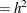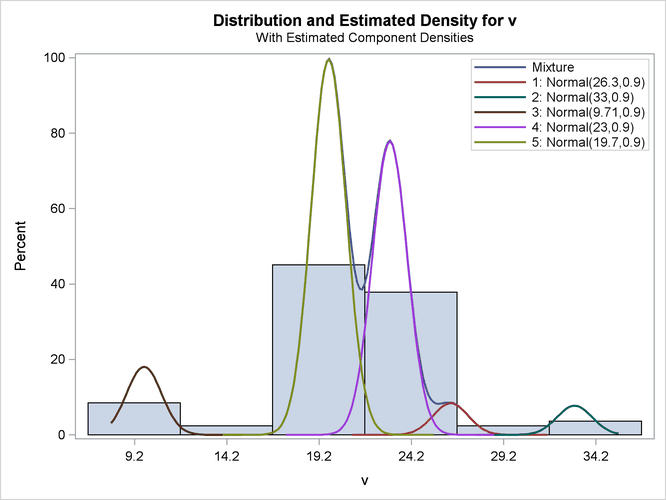#### Comparison with Roeder’s Method

It is important to note that Roeder’s original analysis proceeds in a different manner than the finite mixture modeling presented here. The technique presented by Roeder first develops a best range of scale parameters based on a specific criterion. Roeder then uses fixed scale parameters taken from this range to develop optimal equal-scale Gaussian mixture models.

You can reproduce Roeder’s point estimate for the density by specifying a five-component Gaussian mixture. In addition, use the EQUATE=SCALE option in the MODEL statement and a RESTRICT statement fixing the first component’s scale parameter at 0.9025 (Roeder’s h = 0.95, scale). The combination of these options produces a mixture of five Gaussian components, each with variance 0.9025. The following statements conduct this analysis:

```title2 "Five Components, Equal Variances = 0.9025";
proc fmm data=galaxies;
model v = / K=5 equate=scale;
restrict int 0 (scale 1) = 0.9025;
run;
```

The output is shown in Figure 39.19 and Figure 39.20.

Figure 39.19: Reproduction of Roeder’s Five-Component Analysis of Galaxy Data

 FMM Analysis of Galaxies Data Five Components, Equal Variances = 0.9025

The FMM Procedure

Model Information
Data Set WORK.GALAXIES
Response Variable v
Type of Model Homogeneous Mixture
Distribution Normal
Components 5
Estimation Method Maximum Likelihood

Fit Statistics
-2 Log Likelihood 412.2
AIC (Smaller is Better) 430.2
AICC (Smaller is Better) 432.7
BIC (Smaller is Better) 451.9
Pearson Statistic 82.5549
Effective Parameters 9
Effective Components 5

Linear Constraints at Solution
k = 1   Constraint
Active
Variance = 0.90 Yes

Parameter Estimates for Normal Model
Component Parameter Estimate Standard
Error
z Value Pr > |z|
1 Intercept 26.3266 0.7778 33.85 <.0001
2 Intercept 33.0443 0.5485 60.25 <.0001
3 Intercept 9.7101 0.3591 27.04 <.0001
4 Intercept 23.0295 0.2294 100.38 <.0001
5 Intercept 19.7187 0.1784 110.55 <.0001
1 Variance 0.9025 0
2 Variance 0.9025 0
3 Variance 0.9025 0
4 Variance 0.9025 0
5 Variance 0.9025 0

Parameter Estimates for Mixing Probabilities
Component
Mixing
Probability